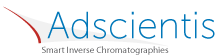## Diffusion Coefficients via IGC

### Quick Start

For stationary phases that are semi-liquid at IGC temperatures, it is straightforward to measure the diffusion coefficients of solvents.

### Diffusion Coefficients

k
φ
h μm
D cm²/s

The Van Deemter equation that controls much of chromatography tells us that the theoretical plate height, H, depends on the flow rate of the gas through the column, u, two constants A and B and a third constant C that depends on the diffusion coefficient of the probe in a polymer stationary phase:

H = A + B/u + Cu

In an IGC experiment, the plate height in a column of length l, with a peak of width d and retention time t, is given by:

H=l.(d/t)²/5.54

Finally, C is related to the thickness of the polymer coating h, and the diffusion coefficient D via:

C = φkh²/[D(1+k)²]

φ is a geometrical factor which can be 8/π² or a more complex factor depending on the packing of the column. The factor k is the "partition coefficient" (tp-tm)/tm where tp is the elution time of the probe and tm is the minimum time through the column.

The app assumes that you have a set of H values at different flow rates and assumes also, that B/u is small so the slope of the curve is C, from which D is derived given the polymer thickness as the other input.

Clearly there are rather too many assumptions in this current version of the app. But as the principle is not well-known, it is better to have a simple app than none at all.The IGC apps are based on the inputs kindly provided by Dr Eric Brendlé of Adscientis who are specialists in IGC measurements.